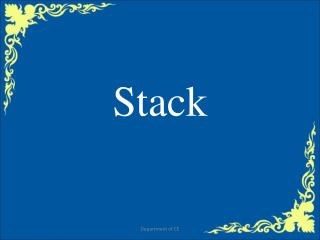DownloadDownload PresentationStack

# Stack

Télécharger la présentation## Stack

- - - - - - - - - - - - - - - - - - - - - - - - - - - E N D - - - - - - - - - - - - - - - - - - - - - - - - - - -
##### Presentation Transcript

1. Stack Department of CE

2. Content • Stack • Stack operation • Push • Pop • Stack Representation • Array • Linked list • Applications Department of CE

3. Stack • A stack is an ordered collection of elements in which insertions and deletions are restricted to one end. The end from which elements are added and/or removed is referred to as top of the stack. Stacks are also referred as “piles” and “push-down lists. The first element placed in the stack will be at the bottom of the stack. The last element placed in the stack will be at the top of the stack. The last element added to stack is the first element to be removed. Hence stacks are referred to as Last-In –First-Out (LIOF) lists. Department of CE

4. Stack Operation • Push: Places an object on the top of the stack. • Pop: Removes an object from the top of the stack and produces that object. • IsEmpty: Reports whether the stack is empty or not. Department of CE

5. Stack Operation Department of CE

6. PUSH push(stack, top, n, item) { if(top=n) then // test whether the stack is full or not { print “stack full”; exit; } else { top=top+1; // top value is incremented by one stack[top]=item; // new item added onto the stack } } Department of CE

7. POP POP (STACK, top, ITEM) { if( top=0) then { print “stack empty”; exit; } else { ITEM=STACK[top] top=top-1; } } Department of CE

8. Array representation of stack • Stacks are represented in the computer memory using array structure or linked list structure. The figure shows the representation using an array namely STACK[N] • N is the size of the stack and top is a pointer variable which holds the index value of the topmost element in the stack. • If the value of top is zero or NULL, the stack is said to be empty. If the value of top is N, then the stack is said to be full. Department of CE

9. Figure N N-1 N-2 N-3 . . . . 6 5 TOP - 4 93 3 58 2 7 1 22 Department of CE

10. Department of CE

11. Linked list representation of stack • This type of representation has more advantages than representing stacks using arrays. They are • It is not necessary to specify the number of elements to be stored in a stack during its declaration. • Insertions and deletions can be handled easily and efficiently. • Linked list representation of stacks can grow and shrink in size without wasting the memory space, depending upon the insertion and deletion that occurs in the list. • Multiple stacks can be represented efficiently using a chain for each stack. Department of CE

12. Application of Stack - Evaluating Postfix Expression (5+9)*2+6*5 • An ordinary arithmetical expression like the above is called infix-expression -- binary operators appear in between their operands. • The order of operations evaluation is determined by the precedence rules and parenthesis. • When an evaluation order is desired that is different from that provided by the precedence, parentheses are used to override precedence rules. Department of CE

13. Application of Stack - Evaluating Postfix Expression • Expressions can also be represented using postfix notation - where an operator comes after its two operands. • The advantage of postfix notation is that the order of operation evaluation is unique without the need for precedence rules or parenthesis.

14. Application of Stack - Evaluating Postfix Expression The following algorithm uses a stack to evaluate a postfix expressions. Start with an empty stack for (each item in the expression) { if (the item is a number) Push the number onto the stack else if (the item is an operator){ Pop two operands from the stack Apply the operator to the operands Push the result onto the stack } } Pop the only one number from the stack – that’s the result of the evaluation

15. Application of Stack - Evaluating Postfix Expression • Example: Consider the postfix expression, 2 10 + 9 6 - /, which is (2 + 10) / (9 - 6) in infix, the result of which is 12 / 3 = 4. • The following is a trace of the postfix evaluation algorithm for the above.

16. END Department of CE